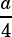Test 5 Section 4 Number 26

To get this one, you first need to know what surface area means. A 3-D figure’s surface area is the sum of the areas of all its faces. In this case we’re dealing with a cube, which has 6 faces of equal area.

If we know this cube has a surface area of, then we can conclude that each of the 6 faces of the cube has an area of.

The faces of a cube are all squares, of course. We know we find the area of a square by squaring one of its side lengths. Knowing the area of a square is, we can take the positive square root to land on its side length:.

This question asks for the perimeter of one face of the cube (i.e., the perimeter of that square we were just working on). If each side has a length of, then the perimeter of the square is.